# Windowing

March 29, 2018

The Fourier series assumes that the signal is periodic in time T. When a signal is digitized into N samples, numbered 0 to N – 1, the Fourier series is based on the assumption that the next sample (numbered N) will have the same value as the first sample (numbered 0). For most vibration signals this is not the case. Therefore, there is usually a discontinuity between the end of the digitized signal and the beginning of the presumed periodic repetition. This causes artificial noise to be generated by the FFT algorithm.

This is illustrated in Figure 2.19 with the simple case of two sine waves: the first exactly fits in the sampled time T = 0.512 sec. with an integer number of cycles (and is therefore periodic in time T); the second is slightly off (and is therefore non-periodic in time T), generating a discontinuity in time and noise in the FFT result. The theoretical PSD of a sine wave is an impulse at its frequency. The discontinuity caused by the non-periodicity of the signal generates spurious amplitudes in the adjacent frequencies called leakage.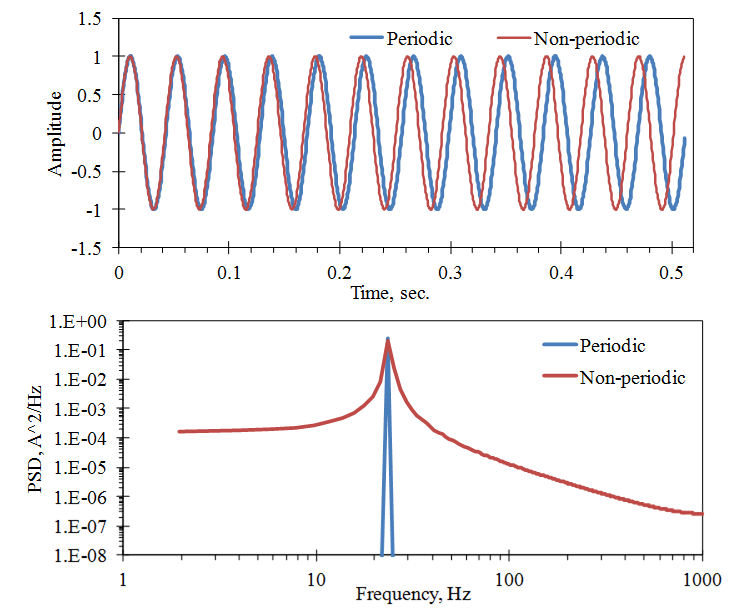Figure 2.19. The effect of periodic and non-periodic signals (in Time T) on the FFT computation. SR = 2000 samples/sec., N = 1024, Periodic: 23.4375 Hz, Non-periodic: 23.9258 Hz.

Note: Here the PSD is plotted as a line function (which is typical because there are so many frequency points) even though it hides the bandwidth aspect of the PSD.

To alleviate the leakage problem (but not totally overcome it) the digitized signal is modified to go to zero at the beginning and the end of the time sample before the FFT is computed. This is called windowing. There are many different window functions but one of the more common is the Hanning window, WH,  shown in Figure 2.20. It is also called the “raised cosine” window because its equation is:

(1)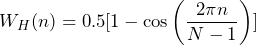Equation 5

Some computer programs, such as MATLAB, have an option to replace the  N – 1  in the denominator of the cosine function with N to make the window periodic in N.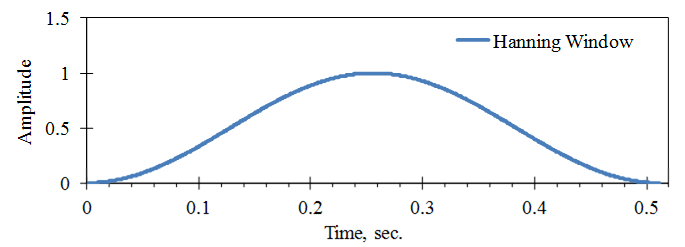Figure 2.20. Hanning window used for a digital sample with SR = 2000 samples/sec. and N = 1024.

Applying this window to the sine waves in Figure 2.19 produces the results shown in Figure 2.21. Several things should be noted. First, the level of the spurious noise generated by the FFT of the non-periodic signal has been greatly reduced for the frequency bands at some distance from the peak frequency, called “remote band” leakage.

However, there is a trade-off. For the frequency bands adjacent to the peak frequency, the PSD level is increased, even for the periodic signal. This is called sideband leakage. It also makes the frequency of the peak less certain. This is discussed further in the next lesson on Frequency Resolution.

Second, the PSD level at the peak frequency has been reduced. Part of this is due to the fact that the window has reduced the mean-square amplitude of the signal in the time sample. This reduction can be corrected by dividing the PSD values by the mean square amplitude of the window function,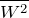, which is 0.375 for the Hanning window and usually included in the PSD computation. Equation 3 can then be revised to:

(2)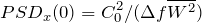(3)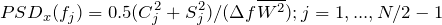Equation 6

The other part of the peak value reduction is due to the leakage which has moved some of the peak value into the sidebands. This must be accounted for by using Equation 4 to sum the mean square values in the frequency bands around the peak instead of just using the peak value.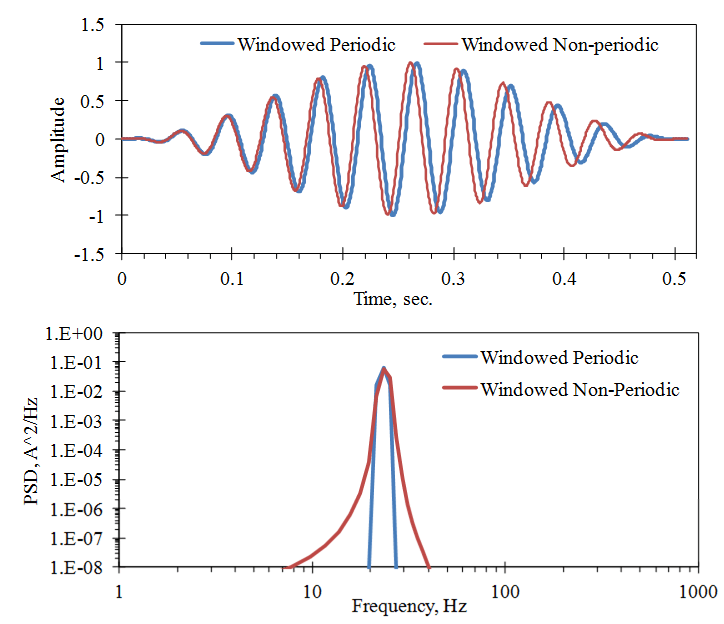Figure 2.21. Effects of the Hanning window on the examples in Figure 2.19.